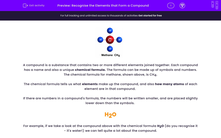# Recognise the Elements that Form a Compound

In this worksheet, students will recognise the elements that make up a variety of compounds.Key stage:  KS 3

Curriculum topic:   Chemistry: Atoms, Elements and Compounds

Curriculum subtopic:   Chemical Symbols and Formulae

Difficulty level:#### Worksheet OverviewA compound is a substance that contains two or more different elements joined together. Each compound has a name and also a unique chemical formula. The formula can be made up of symbols and numbers. The chemical formula for methane, shown above, is CH4.

The chemical formula tells us what elements make up the compound, and also how many atoms of each element are in that compound.

If there are numbers in a compound's formula, the numbers will be written smaller, and are placed slightly lower down than the symbols.

H2O

For example, if we take a look at the compound above with the chemical formula H2O (do you recognise it - it's water!) we can tell quite a lot about the compound.

We can tell that it is made up of hydrogen (H) and oxygen (O). And we can also tell that there are two atoms of hydrogen for every atom of oxygen - that's what the little 2 means after the H.

So for methane, which we saw at the start, the chemical formula is CH4. This means it is made up of two elements - carbon and hydrogen. There is one atom of carbon in methane, and four atoms of hydrogen.

Let's have a look at some common compounds and try some questions about what elements they contain.

It might be helpful to have a paper copy of the periodic table in front of you for these questions.### What is EdPlace?

We're your National Curriculum aligned online education content provider helping each child succeed in English, maths and science from year 1 to GCSE. With an EdPlace account you’ll be able to track and measure progress, helping each child achieve their best. We build confidence and attainment by personalising each child’s learning at a level that suits them.

Get started••••